##### Praxis Core 2023-2024 For DummiesFor the Praxis Core exam, you need to become familiar with many ways to display or represent your data. Using lists, tables, graphs, charts, and plots to represent data is a surefire way to make sure you aren’t tricked by the data. These methods of organizing data can also help you see patterns more readily. In the sections that follow, you become skilled at dissecting and interpreting different types of data representations.

## Tables

When you have gobs of data about a particular subject, you can sort, analyze, and display your data in a table. Tables only work if you have at least two sets of data to be organized into columns and rows.

When working with tables, make sure to pay attention to the title of the table; it helps you understand what data to analyze. Next, notice the column and row titles.

In the following table, the data for the types of flowers and the number of each type of plant in Mary’s flower bed are listed. Make sure to read your question carefully and dissect the data accordingly.
Which ratio compares the number of rose plants to the number of daffodil plants?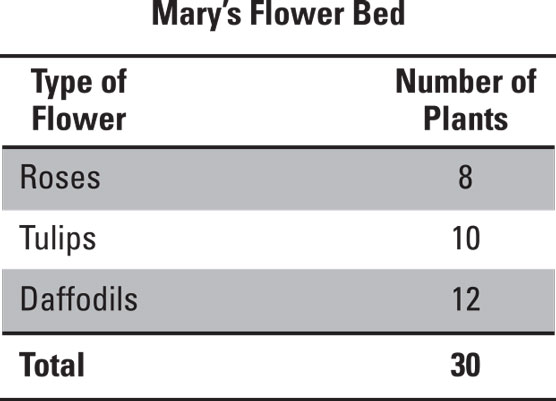A. 3:2

B. 2:3

C. 4:3

D. 5:6

E. 3:4

The correct answer is Choice (B). The ratio of roses to daffodils is 8:12; when factored, the ratio is 2:3. The Praxis Core exam will expect answers in the simplest form.

## Bar graphs and line graphs

A bar graph uses the length of vertical or horizontal bars to represent numbers and compare data. Bar graphs are good to use when your data is in categories. Bar graphs must contain a title, axis labels for the horizontal and vertical axis, scales, and bars that represent numbers.
The following bar graph shows the number of canned goods collected by homerooms at Cardozo Middle School.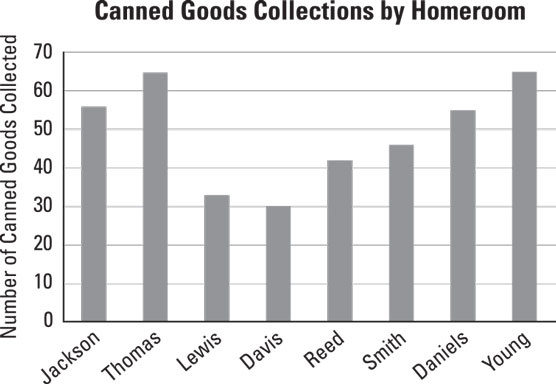Mr. Smith’s homeroom collected more cans than how many other homerooms?

A. 3

B. 4

C. 5

D. 6

E. 7

The correct answer is Choice (A). Use the graph to compare the number of cans collected by each homeroom. According to the lengths of the vertical bars, Mr. Smith’s homeroom collected more cans than Mr. Lewis, Mr. Davis, and Mrs. Reed’s classes.

Line graphs are graphs that show data that is connected in some way over a period of time. Suppose you’re preparing for a statistics test and each day you take a short online quiz to see how you’re progressing. These are the results:

 Day 1 30 percent Day 2 20 percent Day 3 50 percent Day 4 60 percent Day 5 80 percent
After you’ve created a table from your results, display them in a line graph. You can then decide, based on your progress on the practice quizzes, how likely you are to pass your statistics test. What trends do you see in the following graph?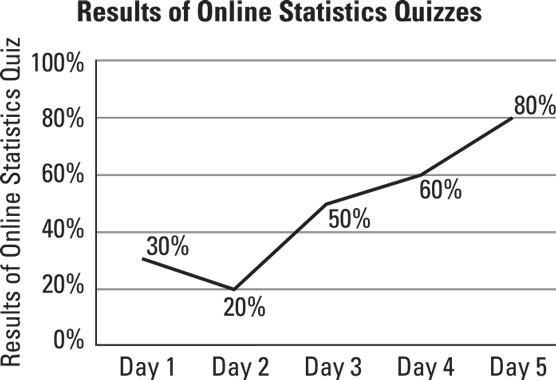The graph indicates that as the days of practicing the online quizzes increase, your score increases; so, you will, more than likely, pass your statistics test.

## Pie charts

Are you ready for a slice of pie? Pie charts are also known as circle graphs. These graphs focus on a whole set of data that is divided into parts. Each category represented in a pie chart is represented by a part, called a sector, of the interior of the circle. The portion of the circle interior a category’s sector takes up is part of what represents the portion of the whole (population, number of items sold, and so on) the category makes up. For example, if a pie chart represents categories of county government spending and 10 percent of the county government spending goes to road maintenance, the category of road maintenance would be labeled in a sector that takes up 10 percent of the interior of the circle. The sector would also have “10%” presented in it, and the sector would be labeled with “Road Maintenance.”
1. Move the decimal point two places to the right and add a percent symbol.
2. Multiply the percent by 360 to get the number of degrees for that slice of your pie chart.
3. Display all the slices together in the pie chart.
The following table shows the conversion of raw data to information that can be used to create a pie chart. The resulting pie chart is shown in the example question.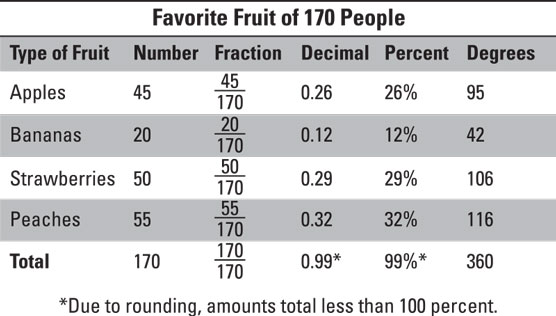When reading a pie chart, the larger the value, the larger the piece of pie!

## Stem-and-leaf plots

A stem-and-leaf plot blossoms into a useful graph when analyzed properly. You usually use this type of graph when you have large amounts of data to analyze. You can analyze data sets such as classroom test results or scores of the basketball team using a stem-and-leaf plot.

Based on place value, each value in your data set is divided into a stem and leaf. What each stem and leaf plot represents is indicated by a Key. Draw a vertical line to separate the stem from the leaf. The leaf is always the last digit in the number. The stem represents all other digits to the left of the leaf. To divide 105 into stem-and-leaf format, you draw a line to separate the stem from the leaf, which indicates a stem of 10 and a leaf of 5.

Say you have the following numbers:

50, 65, 65, 60, 50, 50, 55, 70, 55

The first step is to arrange your data in least-to-greatest order, as follows:

50, 50, 50, 55, 55, 60, 65, 65, 70

Now arrange these numbers vertically in a table:

 Stem Leaf 5 0 0 0 5 5 6 0 5 5 7 0
Key: 5|0 means 50

This arrangement allows you to quickly identify your stems. Your stems in the data set are 5, 6, and 7. You have five data values in the list in the 50s: 50, 50, 50, 55, and 55. The leaves that go along with the 5 stem are 0, 0, 0, 5, and 5. You have three data values in the 60s: 60, 65, and 65. The leaves that go with the 6 stem are 0, 5, and 5. Finally, you have one leaf with a data value of 0 to accompany the stem of 7.

When using a stem-and-leaf plot, you can quickly identify the least and greatest values in the data set (50 and 70), calculate the range (), and calculate the median or middle number (55).

The following data shows the number of people visiting a particular frozen yogurt shop per hour across a 12-hour day.

Hourly customers: 4, 17, 22, 31, 39, 40, 25, 43, 35, 40, 38, 13.

When this data is arranged in a stem-and-leaf plot, you get the following diagram. Use it to answer the questions that follow.

Hourly Customers
 Stem Leaf 0 4 1 3 7 2 2 5 3 1 5 8 9 4 0 0 3
Key: 4|0 means 40
What was the largest number of people that entered the shop during an hour?
The correct answer is 43. Based on the diagram, the highest stem is 4 and the highest leaf in that stem is 3.

## Box-and-whisker plots

Box-and-whisker plots, also known as box plots, show different parts of a data set using a line of numbers that are in order from least to greatest.

A box-and-whisker plot allows you to divide your data into four parts using quartiles. The median, or middle quartile, divides the data into a lower half and an upper half (for more on finding the median, see the later section “Measuring arithmetic mean, median, or mode”). The median of the lower half is the lower quartile. The median of the upper half is the upper quartile. Your data set will contain the following five parts:

• The least value: The smallest value in the data set.
• The lower quartile: The median of the lower half of the data set.
• The median: The median or middle quartile. This divides the data into a lower half and an upper half. The median is the number in the center. If two numbers are in the center, find the average of the two middle values.
• The upper quartile: The median of the upper half of the data set.
• The greatest value: The largest value in the data set.
The following diagram shows how data is dissected using a box-and-whisker plot. To create a box-and-whisker plot, follow the diagram below.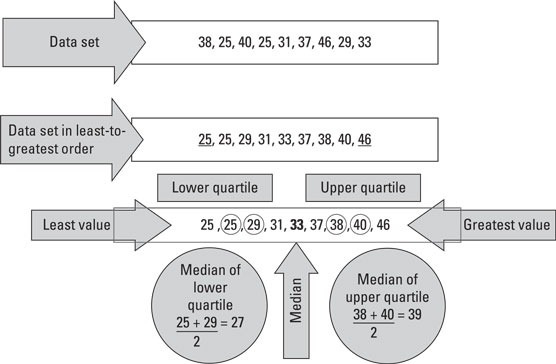The diagram begins with the data set. The data set is then put in least-to-greatest order. Underline the least value and the greatest value in the data set. Then find the median of the entire data set. Remember, when calculating the median, if there are two values in the center of a data set, find their average. The median divides the data set into the lower set and higher set. You must then find the median of the lower set. That is the lower quartile, or Q1. You also must find the median of the upper set. That median is the upper quartile, or Q3. The median of the entire set of data is Q2. The word “quartile” can also be used to refer to a division of numbers marked off by Q1, Q2, Q3, or the highest number in the entire set.

After dissecting the data into the five values, graph the five values on a number line. Draw a box from Q1 to Q3. Draw a vertical line inside the box at Q2. The lines connecting the least and greatest values to the box are called the whiskers.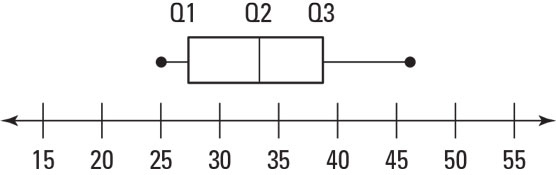Use the following graph to answer the following questions.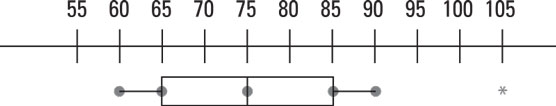This box plot indicates the scores from yesterday’s math test. Approximately what percent of the students did not get above 65%?

A. 25%

B. 50%

C. 65%

D. 75%

E. 85%

The correct answer is Choice (A). The box-and-whisker plot indicates that the lowest score on the test was 60. The median of the lower quartile is 65, so about 25 percent of the students scored lower than 65. It could be exactly 25 percent, depending on the number of students.
What is the median test score from yesterday’s math test?
A. 25%
B. 50%
C. 65%
D. 75%
E. 85%
The correct answer is Choice (D). The median of the box-and-whisker plot is indicated by a line drawn through the center of the box. The value graphed at this point is 75.

## Venn diagrams

“Venn” you need to picture relationships between different groups of things, use a Venn diagram. A Venn diagram is an illustration where individual data sets are represented using basic geometry shapes such as ovals, circles, or other shapes. Simply draw and label two or more overlapping circles to represent the sets you’re comparing. The sets overlap in an area called the intersection. When an item is listed in both sets, it goes in the intersection. If an item doesn’t fit in either set, it falls outside the circles or other shapes.
Use the following graph to answer the following questions.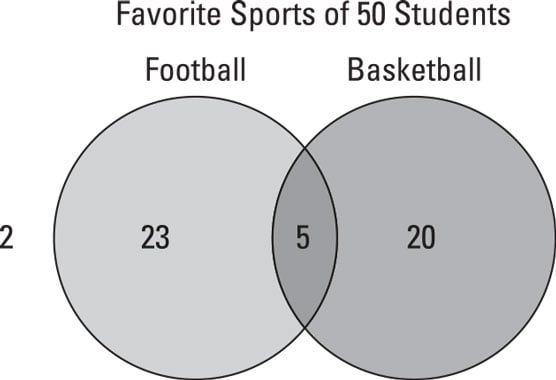Football is the favorite sport of how many students in the Venn diagram?

A. 2

B. 5

C. 23

D. 25

E. 48

The correct answer is Choice (C). In the Venn diagram, 23 students picked football as their favorite sport. This is the only portion of the diagram reserved for football only.
Football and basketball are the favorite sports of how many students?
A. 2
B. 5
C. 23
D. 25
E. 48
The correct answer is Choice (B). Five students in the Venn diagram picked football and basketball. This is the portion of the diagram that football and basketball have in common.
How many students did not choose football or basketball?

A. 2

B. 5

C. 23

D. 25

E. 48

The correct answer is Choice (A). There are two students who did not fall inside the Venn diagram’s circles; therefore, they chose neither football nor basketball.

## Scramble around scatter plots

If you want to determine the strength of your relationship, use a scatter plot. Scatter plots are graphical representations of two variables determining whether a positive, a negative, or no correlation exists. A correlation is a relationship between two variables in which as one increases or decreases, the other one tends to increase or decrease. There is a correlation between time studying and test scores, for example. As time studying increases, test scores tend to increase.

Data from two sets are plotted in scatter plots as ordered pairs (x, y). You can draw three conclusions from scatter plots:

• If the coordinates are close to forming a straight line that rises up from left to right, then a positive relationship or correlation exists.
• If the coordinates are close to forming a straight line (line of best fit) with one variable increasing as the other decreases, then a negative relationship or correlation exists.
• If the coordinates don’t come close to forming a line and are all over the place, then no relationship or correlation exists! Hence, the name scatter plot.
Here are the three types of correlations: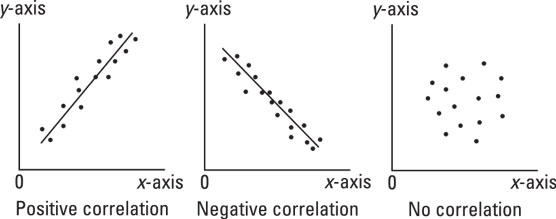Make sure to give your plot a title and make sure to label your axes when you create a scatter plot.

Correlation alone does not prove causation. If a variable tends to increase as another variable increases, for example, it does not mean that the tendency is caused by the other variable. The number of mud puddles tends to become greater when the occurrence of lightning rises. Does an increase in the occurrence of lightning cause the number of mud puddles to get higher? Do the additions of mud puddles cause more lightning? The correlation between the two does not prove that one causes the other, and in fact neither causes the other. An increase in rain causes both.

Also, even when a change in one variable does cause a change in another, that alone does not prove which variable change is causing which. Studies have shown that changes in the closeness of the moon cause shifting of the tides, but the correlation by itself is not the proof. If correlation worked that way, we could just as easily conclude that the shifting of the tides causes major changes in the closeness of the moon.

## Loiter around line plots

If you want to see your mode (the value that occurs the most) pop up quickly, use a line plot. A line plot, also known as a dot plot, allows you to identify the range, mode, and outliers in your data set. Follow these simple steps:
1. Put your data in order from least to greatest.
2. Arrange your data on a number line.
3. Mark each value in the data set with an x or a dot.
Using the following line plot, determine the mode of the data set.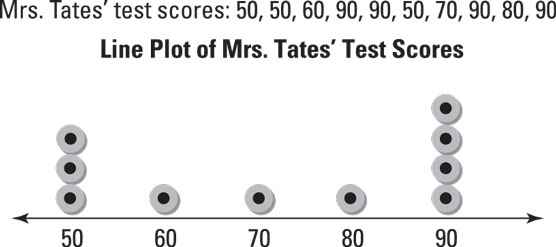A. 50
B. 60
C. 70
D. 80
E. 90
The correct answer is Choice (E). The score of 90 appears in the data set the most.